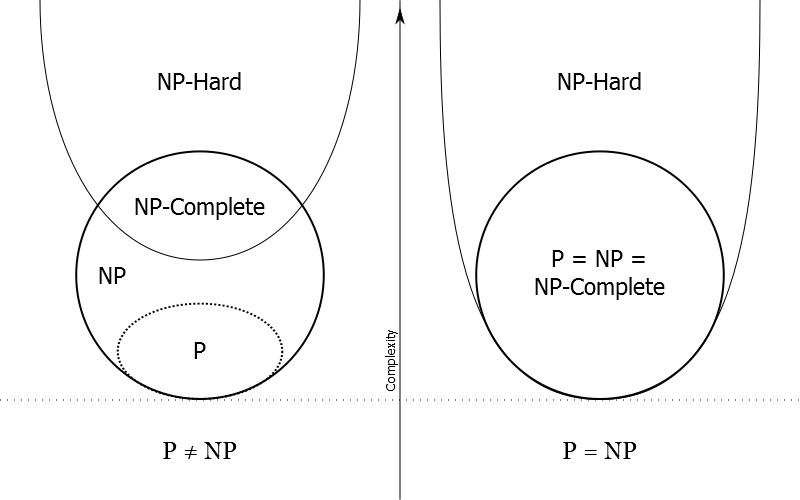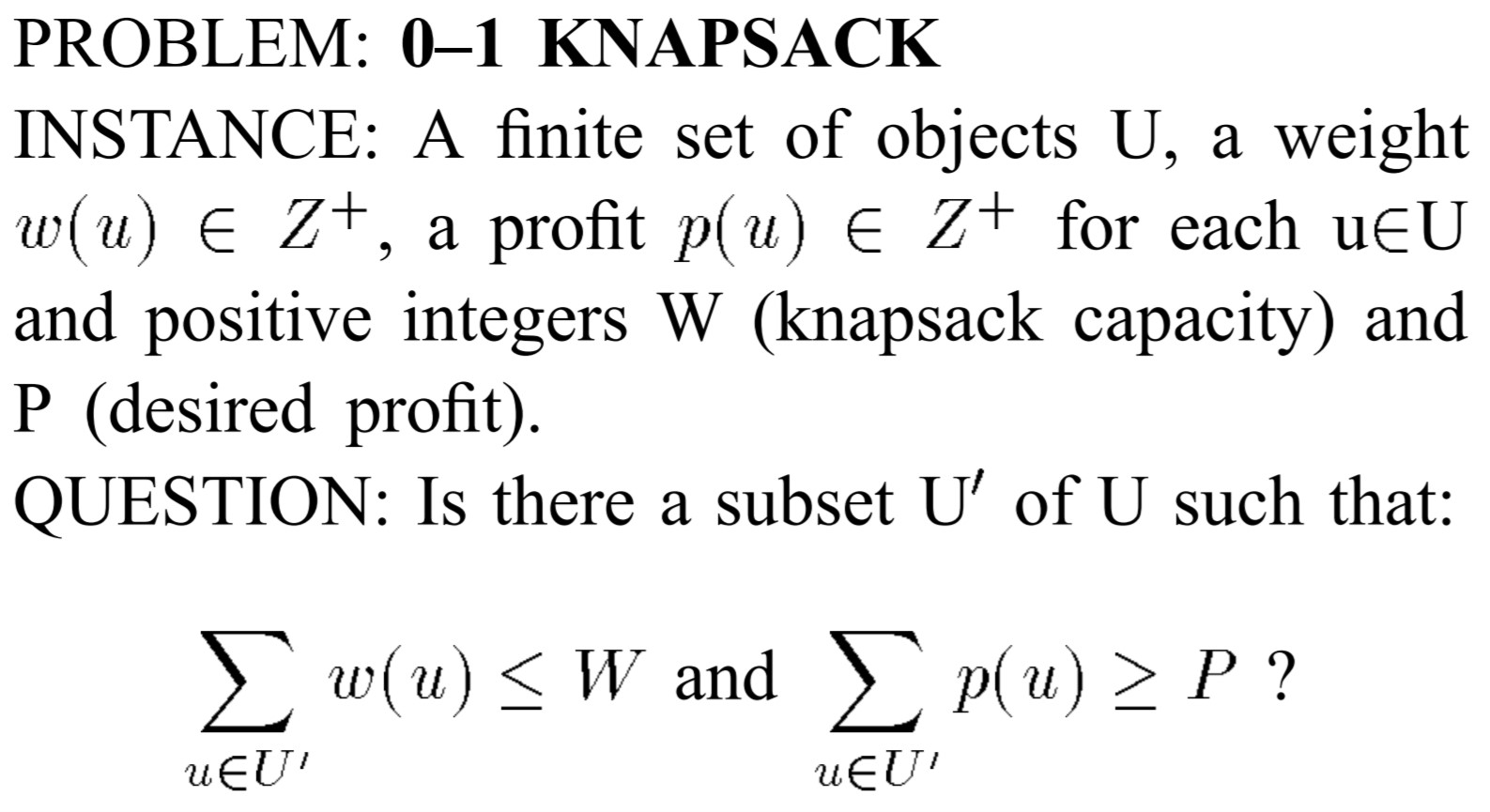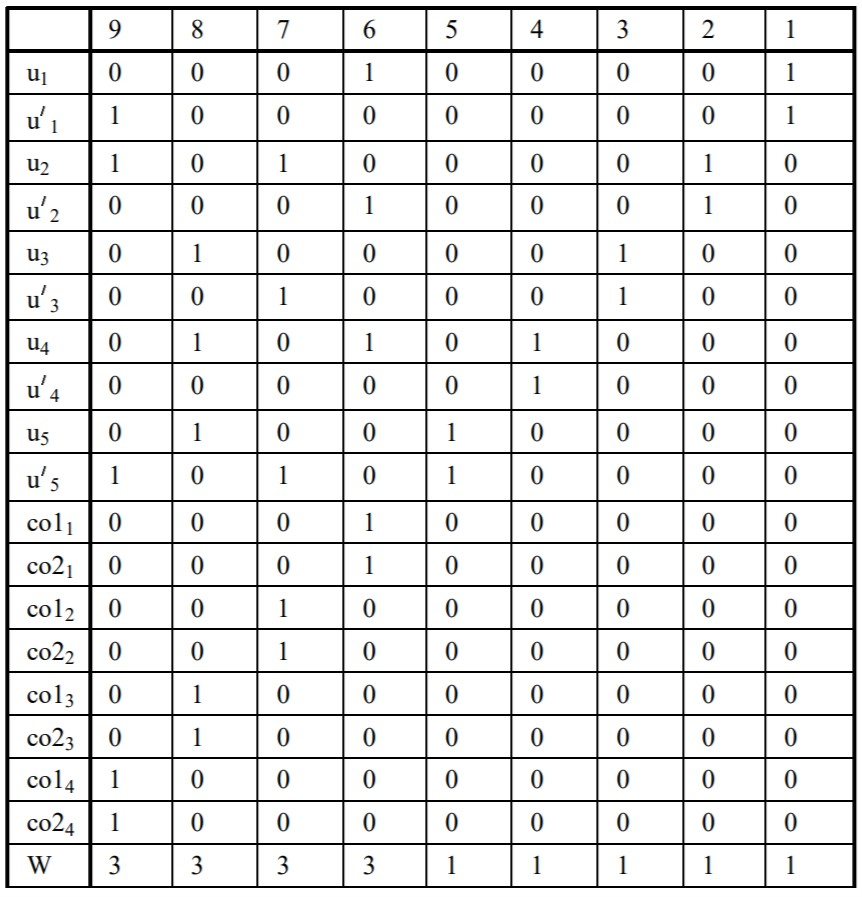# 计算复杂性理论

### 计算复杂性理论

##### NP-C问题(NP-Complete)

a) 该问题是一个NP问题
b) 所有属于NP的问题都可归约成该问题

a) 证明给定该问题的一个解，可以在多项式时间验证该问题
b) 可以将一个已知的NP-C问题规约到该问题(已经证明的NPC问题：卡普的21个NPC问题或者A compendium of NP optimization problems)

Cook定理(1971) 可满足问题属于NP-C

##### NP-Hard### 基本概念

##### 规约(reduction)

• 问题A能够规约为问题B
a) 一个能求解问题B的算法一定可以用来求解问题A（以子问题的形式）
b) 求解问题A的难度一定不会比求解问题B的难度大（这里的难度大指的是求解过程需要更多的计算、存储资源等）。——>可以从侧面证明，如求解A的难度更大，而由于A可以规约为问题B，则可以用求解问题B的算法来求解问题A，则求解A的算法可以替换为一个难度更低的算法

• 规约具有传递性：A可以规约为B，B能规约为C，则A一定可以规约为C

* 把P的输入转化到Q的输入；
* 把Q的输出转化到P的输出。

### 3SAT

3SAT问题定义如下：

* x_i是布尔变量（Variable）
* 一个布尔变量x_i或它的否定形式\bar{x_i}是文字（literal）
* C_i为子句，一个子句包含3个文字（literal）

X={x_1,x_2,x_3},C={C_1,C_2,C_3},C_1=x_1\cup \bar{x_2}\cup x_3,C_2=\bar{x_1}\cup \bar{x_2}\cup x_3,C_3=\bar{x_1}\cup x_2\cup \bar{x_3}

3SAT\in NPC

### 0-1背包问题

0-1 背包问题（0-1 Knapsack）在数学上的定义如下：0-1背包问题本质上是一个优化问题，为了证明0-1背包问题是一个NP-C问题，我们首先引入0-1背包问题对应的判定问题（记作0-1 Knapsack Fill ）

1. 证明0-1 Knapsack Fill \in P问题

1. 将一个已知的NP-C问题规约到0-1 Knapsack Fill问题(多项式时间内)
证明3SAT<=0-1 Knapsack Fill

• 对于一个3SAT问题，设X={x_1,x_2,\cdots,x_n},|X|=n，有一组子句C={C_1,C_2,\cdots,C_m},|C|=m,C=C_1\cap C_2\cap\cdots \cap C_m
• 对于0-1 Knapsack Fill问题，存在一组物品U和背包容量W，我们希望得到结论：当且仅当C可满足时，存在物品集合的子集U^{‘}\in U 使得\sum_{u\in U^{‘}} w(u)=W

为了将3SAT规约到0-1 Knapsack Fill，做出以下定义：

• 对于每个布尔变量x_i，对应u_i和\bar{u_i}
• 对于每个子句c_j，定义两个补偿对象（compensating objects）co1_j和co2_j
• (n+m)长度的3进制数表示物品重量w(u)，其中第i个数字与布尔变量x_i相关；第(n+j)个数字，与子句c_j相关。
• 对于u_i和\bar{u_i}
• 最右边n个数字:第i个数字为1，其余数字为0
• 最左边m个数字:如果x_i(与u_i相关)或者\bar{x_i}(与\bar{u_i}相关)在子句c_j中出现，则第n+j个数字为1，其余数字为0
• 对于co1_j和co2_j
• 最右边n个数字:全为0
• 最左边m个数字（用来标识对应的子句c_j）:第n+j个数字为1，其余数字为；co1_j和co2_j的重量相同
• 背包容量W
• 最右边n个数字:全为1
• 最左边m个数字:全为3

通过上述转化过程，我们将一个3SAT问题归约到了0-1 Knapsack Fill实例（显然该过程是在多项式时间内，因为每一步的转化过程都是确定的），该实例有以下特点：

• U={u_i,\bar{u_i}: 1\leq i\leq n}\cup{co1_j,co2_j: 1\leq j \leq m}
• w(u_i)由(n+m)个二进制数组成
• i个数字为1
• 当且仅当x_i在子句c_j中出现时，第n+j个数字为1
• 其余所有数字均为0
• w(\bar{u_i})由(n+m)个二进制数组成
• i个数字为1
• 当且仅当\bar{x_i}在子句c_j中出现时，第n+j个数字为1
• 其余所有数字均为0
• w(co1_j)=w(co2_j)由(n+m)个二进制数组成
• n+j个数字为1
• 其余所有数字均为0
• 背包容量W(n+m)长度的二进制数
• 最右的n个数为1
• 最左的m个数为3

对于任意一个3SAT问题，我们都可以通过上述过程转化为一个0-1 Knapsack Fill问题，为证明0-1 Knapsack Fill问题的复杂性，只需证明：当且仅当C可满足时，存在物品集合的子集U^{‘}\in U 使得\sum_{u\in U^{‘}} w(u)=W

• 先证明存在物品集合U^{‘}\in U使得\sum_{u\in U^{‘}} w(u)=W时，C可满足
• 假设已得到部分物品集合U^{‘}\in U使得\sum_{u\in U^{‘}} w(u)=W
• 最右边的n个数字全为1：保证u_i,\bar{u_i}有且仅有一个出现在集合U^{‘}(否则第i个数字的值为0或2，与假设冲突)——>可以得到一组3SAT中布尔变量的赋值，记作v
• 如果u_i\in U^{‘},则有x_i^{v}=1
• 如果\bar{u_i}\in U^{‘},则有\bar{x_i}^{v}=1或者x_i^v=0
• 最右边的m个数字均为3：保证每个子句c_j均是可满足的，即C可满足
• 如果co1_j,coj_2均不属于U^{‘}，有\sum_{u\in U^{‘},u=u_i or u=\bar{u_i}} w(u)=3。所以子句c_j中的布尔变量均为1，所以每个子句均可满足
• 有且仅有co1_j,coj_2中的一个属于U^{‘}，，有\sum_{u\in U^{‘},u=u_i or u=\bar{u_i}} w(u)=2。所以子句c_j中有两个布尔变量为1，所以每个子句均可满足（合取范式）
• co1_j,coj_2均属于U^{‘}，，有\sum_{u\in U^{‘},u=u_i or u=\bar{u_i}} w(u)=1。所以子句c_j中有一个布尔变量为1，所以每个子句均可满足（合取范式）
• 证明当C可满足时，存在物品集合的子集U^{‘}\in U 使得\sum_{u\in U^{‘}} w(u)=W
• 与上述证明过程类似，这里不再赘述

通过上述过程，我们有3SAT<=0-1 Knapsack Fill

• 输入过程：x_i,\bar{x_i}与u_i,\bar{u_i}对应；x_i,\bar{x_i}是否在子句c_j中出现由第(n+j)个数字标识，结合而成的(n+m)个数字表示物品重量
• 输出过程：当且仅当C可满足时，存在物品集合的子集U^{‘}\in U 使得\sum_{u\in U^{‘}} w(u)=W

给出一个3SAT<=0-1 Knapsack Fill的例子：

3SAT:
X={x_1,x_2,x_3,x_4,x_5},C={c_1,c_2,c_3,c_4},c_1={x_1,\bar{x_2},x_4},c_2={x_2,\bar{x_3},\bar{x_5}},c_3={x_3,x_4,x_5},c_4={\bar{x_1},x_2,\bar{x_5}}
对应的0-1 Knapsack Fill问题：
U={u_1,\bar{u_1},u_2,\bar{u_2},u_3,\bar{u_3},u_4,\bar{u_4},u_5,\bar{u_5},co1_1,co2_1,co1_2,co2_2,co1_3,co2_3,co1_4,co2_4},W=333311111.
物品集合U中物品对应重量为：* 可以从侧面给出不严谨证明：0-1 knapsack是一个优化问题，如果能在多项式时间内验证一个解，相当于能在多项式时间内求出该优化问题的最优解

## 2 thoughts on “计算复杂性理论”

1.yuanxin说道：

你好，“NP∈P”是笔误吗

1.akeeper说道：

是的，感谢指正，已修改